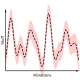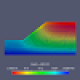# A Rigid Bar Walks Into a Bar

OpenSees has two `rigidLink` commands that enforce constraints between a primary node (`pNode`) and a secondary node (`sNode`).

```ops.rigidLink('-beam',pNode,sNode)
```

The `beam` option works well, enforcing linear kinematic constraints as if the two nodes were connected by a beam of infinite axial and flexural stiffness. The `bar` option should give constraints assuming only infinite axial stiffness.

But, like in Italy, the `bar` may not be what you think it is.

Consider the model shown below. Element 1 is an `elasticBeamColumn` element while element 2 is an elastic `truss` element.

Due to a vertical load at node 3, with realistic member properties that give naturally high axial stiffness, element 2 will rotate about the instantaneous center of rotation (ICR) shown in the figure, and push element 1 to the left, as shown in the deformed shape below, plotted using `opsvis`.

Now, let’s make the high axial stiffness of element 2 official by using a rigidLink `bar` constraint. There should not be any significant difference in response because element 2 already has high axial stiffness.

However, when we apply this constraint to the model, the deformed shape is totally different.

Now element 2 does not rotate, i.e., the ICR is at infinity. The vertical load transfers directly into axial compression of element 1. Note that the scale factor on the deformed shape is huge.

In addition, the support reactions of the model do not satisfy equilibrium. You have to include the “reactions” from node 2 in order to make global equilibrium work out.

So what’s the problem? No, we did not daisy chain the single point constraints at node 3 with the rigid link multi-point constraint.

The problem is the rigid link `bar` in OpenSees constrains the translations at each node to be equal, i.e., the global X-displacements of the primary and secondary nodes are equal and the global Y-displacements of the primary and secondary nodes are also equal. Same thing for Z-displacements in 3D.$u_1=u_4$,$u_2=u_5$, and$u_3=u_6$

The constraint equation for a rigid bar should be$\Delta X(u_4-u_1)+\Delta Y(u_5-u_2)+\Delta Z(u_6-u_3)=0$

where$\Delta X=X_J-X_I$ is the difference in global X-coordinates of nodes I and J. Same for$\Delta Y$ and$\Delta Z$. From this constraint equation we can select one secondary DOF, e.g., u1$u_1=u_4 - (\Delta Y/\Delta X)(u_5-u_2) - (\Delta Z/\Delta X)(u_6-u_3)$

Of course we have to check if$\Delta X$ is zero. If so, we can rewrite the equation with u2 or u3 on the left-hand side.

But there’s really no easy way to pull off this constraint equation in OpenSees.

The MP_Constraint class, from which `rigidLink`, `rigidDiaphragm`, `equalDOF`, etc. are constructed, assumes the primary and secondary DOFs are at unique nodes. But with the rigidLink `bar` option, the secondary DOF will be at the same node as one of the primary DOFs.

It’s possible to deal with this case by overloading the MP_Constraint constructor. But no one is beating down the bar doors for this functionality. Just be careful when using the rigid link `bar` command–in fact, I don’t recommend you use the command at all. The `beam` is fine, just not the `bar`.

And if you do want a stiff drink at an Italian bar, ask for a caffè corretto.

## 4 thoughts on “A Rigid Bar Walks Into a Bar”

1.Luigi Caglio says:

That’s surprising! I always assumed it was the same as a stiff truss element. So it’s some “use at your own risk” business just like caffè corretto 🙂

Liked by 1 person

1.Michael H. Scott says:

Yes, or don’t use at all!

Liked by 1 person

2.Sukumar Baishya says:

Dear Professor Scott,
I always admire not only your deep insight about OpenSees and structural Engineering, but i am more a fan of your great sense of humour and your love of music too… Many a times, many of us tend to forget that we are primarily human.. Cheers… 🙂

Liked by 2 people

1.Michael H. Scott says:

Thank you for the kind words, Sukumar! Writing a blog is much more human than other writing we do as academics.

Liked by 1 person

This site uses Akismet to reduce spam. Learn how your comment data is processed.